# Writing equations in Microsoft Word

A student asked me about using LaTeX the other day, and I stated that it is a bit of a hassle for journal articles in our field, so I have begun to use it less. Most of the journals in my field (criminology and criminal justice) make it difficult to turn in an article in that format. Many refuse to accept PDF articles outright, and last time I submitted a LaTeX file to JQC (a Springer journal) that would not compile I received zero help from staff over a month of emails, so I just reformatted it to a Word document anyway. Last time I submitted a LaTeX document to Criminology a reviewer said it probably had typos — without pointing out any of course. (FYI folks, besides doing the obvious and pointing out typos if they exist, my text editor has a spell checker same as Word to highlight typos.) Besides this, none of my co-workers use LaTeX, so it is a non-starter for when I am collaborating. I did my dissertation in LaTeX, and I would do that in LaTeX again, but smaller articles are not a big deal.

The main nicety of LaTeX are math equations. I don’t do too heavy of math stuff, and I have figured out the Microsoft Word equation editor enough to suit most of my needs. So here are a set of examples for many of the use cases I have needed to use in journal articles. I also have this in a Word (docx) document and a PDF for handy reference. Those have a few references I gathered from the internet, but the best IMO is this guys blog (who I think is a developer for Word) and this document authored by the same individual.

One of the things to note about the equation editor in Word is that you can type various shortcuts and then they will be automatically converted. For example, you can type \gamma, hit the space bar, and then the equation will actually change to showing the gamma symbol. So there are some similarities to LaTeX. (Another pro-tip, to start an equation in Word you can press Alt=.) In the subsequent examples I will use <space> to represent hitting the space bar, and there are other examples of using <back> (for the left arrow key) and <backspace> for the backspace button.

## Greek characters, subscripts and superscripts

If you type

log<space>(\lambda) = \beta_0<space> + \beta_1<space>(X) + \beta_2<space>(X^2<space>)

you get: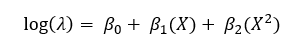## Accents

For these you need to hit the space key twice, so

x\hat<space><space> = y\bar<space><space>

turns into: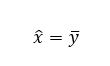## Expected value and variance

For the equivalent of \mathbb in LaTeX, you can do

\doubleV<space>(X)= \doubleE<space>(X)^2<space> + \doubleE<space>(X^2<space>)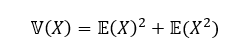## Plain text within equation

To do plain text within an equation, equivalent to \text{*} in LaTeX, you can use double quotes. (Note that you do not need a backslash before “log”.) So

Y = -1\cdot<space>log<space>("Property Crime"<space>) + (not pretty text)

looks like: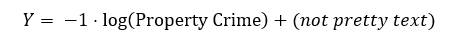## Sum and product

To get the product symbol is simply \prod<space>, and here is a more complicated example for the sum:

n^-1\cdot<space>\sum^n_(i=1)<space>x_i<space>= x\bar<space><space>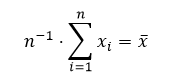## Square root

Square roots always cause me trouble for how they look and kern (both in LaTeX and Word). Here is how I would do an example of Euclidean distance,

d_ij<space>=\sqrt<space><space><back>(x_i-x_j)^2+(y_i-y_j)^2<space>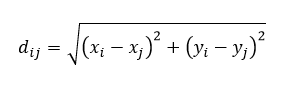## Fractions

The big (stacked) fraction is simple, but I had to search for a bit to find how to do inline fractions (what Word calls “linear”). So here back slash followed by forward slash does the inline fraction:

1/n = 1\/n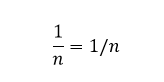## Numbering an equation

I’ve seen quite a few different hacks for numbering equations in Word. If you need to number and refer to them in text often, I would use LaTeX. But here is one way to do it in Word.

E = mc^2#(30)<enter>

produces below (is it just me or does this make the equation look different than the prior ones in Word?):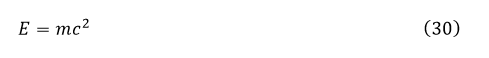## Multiple lines of equations

For a while I did not think this was possible, but I recently found examples of multiline equations (equivalent to \align in LateX). The way this works is you place a & sign before the symbols you want to line up (same as LaTeX), but for Word to split a line you use @. So if you type

\eqarray(10x&=4y@5x&=2y)\eqarray<space><backspace>

you will get: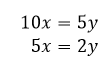Have any more good examples? Let me know in the comments!

The other nicety of LaTeX is formatting references — you are on your own though for that in Word though.

1.#### ses17

/  October 5, 2018

Thanks! It saved my time!
p.s. the variance formula seems wrong 😉

•#### apwheele

/  October 5, 2018

Good call, will need to update that. Thank you!

2.#### Geber

/  January 21, 2019

This is what I’m looking for Multiple lines of equations, but how if we want to add equation number each line?

•#### apwheele

/  January 21, 2019

Sorry, not sure how to do that! Post a comment back if you are able to figure it out.

•#### Ruben

/  August 5, 2019

A bit late, the only way that I’ve found is by using Tables, sadly the equations and their numbers have different heights, so manual spacing is necessary.